A multilevel in space and energy solver for multigroup diffusion eigenvalue problems

Đăng ngày 7/3/2019 10:05:25 PM | Thể loại: | Lần tải: 0 | Lần xem: 10 | Page: 10 | FileSize: 0.63 M | File type: PDF
A multilevel in space and energy solver for multigroup diffusion eigenvalue problems. n this paper, we present a new multilevel in space and energy diffusion (MSED) method for solving multigroup diffusion eigenvalue problems. The MSED method can be described as a PI scheme with three additional features: (1) a grey (one-group) diffusion equation used to efficiently converge the fission source and eigenvalue, (2) a space-dependent Wielandt shift technique used to reduce the number of PIs required, and (3) a multigrid-in-space linear solver for the linear solves required by each PI step.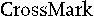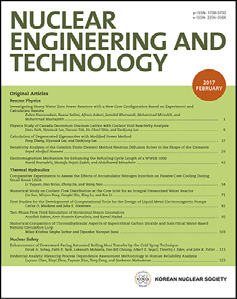Contents lists available at ScienceDirect
Nuclear Engineering and Technology
journal homepage: www.elsevier.com/locate/net
Original Article
A multilevel in space and energy solver for multigroup diffusion
eigenvalue problems
Ben C. Yee*, Brendan Kochunas, Edward W. Larsen
Department of Nuclear Engineering and Radiological Sciences, University of Michigan, Ann Arbor, MI 48109, USA
a r t i c l e
i n f o
a b s t r a c t
Article history:
In this paper, we present a new multilevel in space and energy diffusion (MSED) method for solving
Received 2 June 2017
Received in revised form
14 July 2017
Accepted 27 July 2017
Available online 5 August 2017
multigroup diffusion eigenvalue problems. The MSED method can be described as a PI scheme with three
additional features: (1) a grey (one-group) diffusion equation used to efciently converge the ssion
source and eigenvalue, (2) a space-dependent Wielandt shift technique used to reduce the number of PIs
required, and (3) a multigrid-in-space linear solver for the linear solves required by each PI step. In
MSED, the convergence of the solution of the multigroup diffusion eigenvalue problem is accelerated by
Keywords:
Multigroup diffusion
Multilevel
Eigenvalue
performing work on lower-order equations with only one group and/or coarser spatial grids. Results
from several Fourier analyses and a one-dimensional test code are provided to verify the efciency of the
MSED method and to justify the incorporation of the grey diffusion equation and the multigrid linear
solver. These results highlight the potential efciency of the MSED method as a solver for multidi-
mensional multigroup diffusion eigenvalue problems, and they serve as a proof of principle for future
work. Our ultimate goal is to implement the MSED method as an efcient solver for the two-
dimensional/three-dimensional coarse mesh nite difference diffusion system in the Michigan parallel
characteristics transport code. The work in this paper represents a necessary step towards that goal.
© 2017 Korean Nuclear Society, Published by Elsevier Korea LLC. This is an open access article under the
1. Introduction
from existing ideas (multigrid-in-space  and two-grid in energy
) as well as new ideas (space-dependent Wielandt shift ). The
The multigroup diffusion eigenvalue problem is an approxima-
three primary components of MSED are: (1) a grey(one-group)
tion to the multigroup neutron transport eigenvalue problem that
diffusion equation, used to converge the eigenvalue and ssion
is widely used for reactor physics simulations. The solution is
source, (2) a space-dependent Wielandt shift, used to reduce the
frequently used to accelerate the source iteration procedure for
number of power iterations (PIs) required for convergence, and (3)
solving neutron transport problems via methods such as coarse
a multigrid-in-space solver, used to solve the xed-source grey and
mesh nite difference (CMFD) . Although solving a diffusion
multigroup diffusion linear systems.
problem requires signicantly fewer computational resources than
The MSED method can be viewed as an extension of the CMFD
solving a transport problem, this cost is still not trivial. Many
method. In CMFD, the convergence of a higher-order (more un-
transport codes that use CMFD-like procedures (e.g., the Michigan
knowns) transport or nodal diffusion system is accelerated by
parallel characteristics transport (MPACT) code ) have a CMFD
leveraging a lower-order diffusion system. In MSED, this lower-
eigenvalue problem with hundreds of millions of unknowns, and
order diffusion system is itself accelerated by simpler diffusion
obtaining solutions to this problem constitutes a large portion of
equations with even fewer unknowns. Fig. 1A provides a visuali-
the computational effort.
zation of this hierarchy. Alternatively, the MSED method can be
In this work, we introduce a new multilevel in space and energy
viewed as an extension of the multigrid method to nonspatial
diffusion (MSED) method for solving the multigroup diffusion
variables. Fig. 1B provides a visualization of the changes in spatial
eigenvalue problem. This is a multicomponent method that draws
and energy grid sizes in the MSED iteration scheme. These two
gures are further explained in Sections 2 and 3.
Of the methods that have been developed for reactor physics
* Corresponding author.
simulations, the multilevel coarse mesh rebalance (MLCMR) and
E-mail addresses: bcyee@umich.edu (B.C.
Yee),
multilevel surface rebalance (MLSR) methods developed by van
1738-5733/© 2017 Korean Nuclear Society, Published by Elsevier Korea LLC. This is an open access article under the CC BY-NC-ND license (http://creativecommons.org/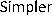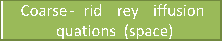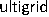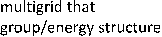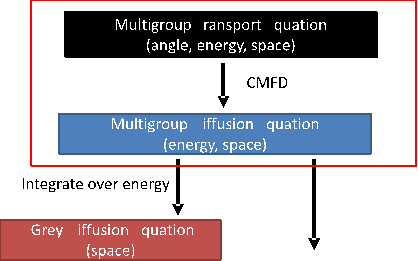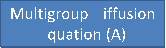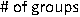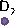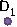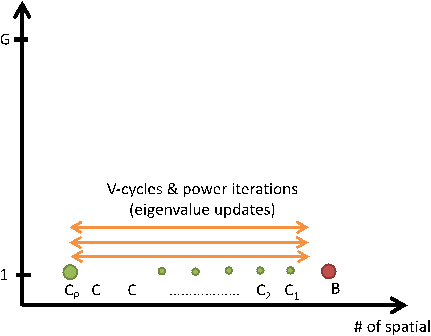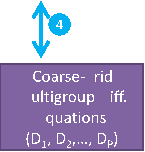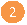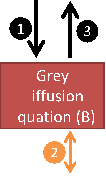^
^
1126
B.C. Yee et al. / Nuclear Engineering and Technology 49 (2017) 1125e1134
t
e
e
d
d
e
g
d
e
m
e
d
d
e
m
d
g m
e
d
g
e
g
e
g
g
d
(A)
(B)
Fig. 1. Each gure provides an overview of the MSED iteration procedure. (A) The hierarchy of the equations in MSED is shown, with a scale on the left describing the relative
complexity of these equations (i.e., the number of unknowns). The higher (red) dashed box encloses the equations used in the CMFD method, while the lower (green) dashed box
encloses the equations used in the MSED method. (B) An MSED iteration is broken up into four steps, and the changes in the energy and spatial grid sizes at each step are visualized.
Geemert and others [6e8] are the most similar to MSED. The
found that the MSED method with a grey equation already per-
MLCMR and MLSR methods are techniques for nodal diffusion
forms very well. Our Fourier analysis and numerical results indicate
problems, which leverage solutions from a series of lower-order
that the MSED method, as described in this paper, has a spectral
coarse-grid one-group diffusion equations in order to minimize
radius of ~0.1. Because of this low spectral radius and the fact that a
the number of iterations required on the full higher-order nodal
grey system is simpler (easier to implement and solve) than a two-
diffusion equations. In this sense, the approaches taken by the
group system, we have not yet been compelled to study the use of a
MSED method and the MLCMR/MLSR methods are similardboth
two-group system in the MSED method. Recent work by Cornejo
methods minimize the computational effort required by shifting
and Anistratov, however, has shown that additional energy grid(s)
iterations from higher-order equations to lower-order equations
between one group and G groups [12,13] can provide tangible im-
that are less costly to solve. The MSED method is also similar to
provements in the runtime, and it may be possible to use a similar
multilevel CMFD methods [1,9,10]. In these cited works, the
strategy to improve the MSED algorithm. In future work, we will
multigroup CMFD equations are themselves accelerated by two-
assess the potential benet of both introducing an extra two-group
group CMFD equations.
system to MSED and replacing the one-group system in MSED with
The MSED method draws from elements of both the rebalance
a two-group system.
and multilevel CMFD concepts, but there are several important
Another motivation for the development of the MSED method is
distinctions. First, the CMFD problem motivating the development
to reduce the reliance of MPACT on black-boxKrylov linear
of MSED is orders of magnitude larger (in terms of the number of
solvers. In recent years, many diffusion and CMFD codes have
unknowns and the number of processors used) than any of the
become increasingly reliant on Krylov methods for solving their
applications in the works referenced above. Second, unlike the
linear systems. These methods are generallyeasy to implement due
MLCMR/MLSR techniques, MSED operates on a diffusion/CMFD
to their availability in various linear algebra libraries such as PETSc
system in which the unknowns representing the neutron net cur-
. They perform reasonably well when compared to other
rent have already been eliminateddthis simplies the process of
frequently used linear solvers such as SOR or GausseSeidel. How-
collapsing onto coarser spatial/energy grids. Third, whereas the
ever, many Krylov solvers (GMRES in particular) require a signi-
multilevel CMFD techniques use ux-weighted cross-sections and
low-order consistency factors(commonly denoted by D, and
cant amount of memory and may not be well-suited for high-
performance computing applications where memory is a limiting
sometimes described as a driftvector/term) to generateits group-
resource. Moreover, Krylov methods generally do not account for
collapsed equations, the MSED method uses both ux-weighted
the physics and structure of the problem being solved, and their
cross-sections and ux-weighted diffusion coefcients, and does
not need additional consistency factorslike the D in the multi-
convergence is typically slow for large problems unless a good
physics-based or problem-dependent preconditioner is applied to
level CMFD methods. In this sense, the collapse in energy in MSED
the system. In manycases, such a preconditioner may not be known
is similar to that of the MLCMR/MLSR methods. Another example in
and, even if one exists, constructing the preconditioner and
which the diffusion coefcients are group-collapsed via ux-
applying it to the linear system may require a signicant compu-
weighting can be found in Schunert et al. ; there, the SN FEM-
tational effort. The approach taken by MSED is fundamentally
discretized transport equations are accelerated by coarse-group
different from those taken by the Krylov methods. Whereas Krylov
FEM-discretized diffusion equations. However, the coarsening in
methods are applicable to general linear systems, MSED is opti-
space in MSED differs from all of the aforementioned exam-
mized only for multigroup diffusion/CMFD eigenvalue problems.
plesdthe spatial variable in MSED is collapsed using a standard
MSED leverages our knowledge of the physics and structure of the
multigrid approach in which coarse-grid equations are error
multigroup diffusion problem and is designed to exploit the unique
equations rather than approximations to the original system.
features of this problem.
Moreover, this collapse in MSED is performed on both the grey and
In the following sections, we provide an overview of the theory
the multigroup equations, as illustrated by Fig. 1B.
for the three components of MSED, describe the full algorithm, and
Lastly, we note that, like the MLCMR/MLSR methods, MSED uses
present results from our Fourier analysis and one-dimensional (1D)
a one-group (grey) low-order equation rather than a two-group
test code. This paper should be viewed as an initial report for the
equation. Although the referenced multilevel CMFD methods all
development of the MSED method, and the work presented in this
use a two-group structure as their coarsest energy grid, we have
paper is a necessary initial step towards our ultimate goal off  f f  f
2 2 2 2
1
  D
D
D
D
D
x
x
x
2 2
j
1 1
X
2 2
G
ðlÞ
  D f
D þ S
D
D
D
x
x
x
2 2
j
1 1
2 2
X
X
n
S f
l
l
;
¼
n
S f
2
1
 
1
lþ
1
 
j;g
0
(l)
1
1
j,g
B.C. Yee et al. / Nuclear Engineering and Technology 49 (2017) 1125e1134
1127
implementing MSED as a solver for the multidimensional CMFD
problem in MPACT. As this development progresses and as we learn
more about the performance of the MSED method, it is likely that
changes will be made to the algorithm so that it performs optimally
in parallel on both two-dimensional (2D) and three-dimensional
(3D) CMFD problems.
" ðlþ1Þ ðlþ1Þ ðlþ1Þ ðlþ1Þ #
jþ1;g j;g j;g j1;g
jþ1;g j1;g
jþ2 j2
   
lþ1 G lþ1
þSt;j;gfj;g  Ss0;j;g0 /gfj;g0
g0¼1
(4a)
2. Theory
The 1D multigroup diffusion equation (the blue box in Fig. 1A),
discretized using a second-order nite-difference scheme, is given
¼ lðlÞcj;g X nSf;j;g0 fj;g0 ;
g0¼1
by:
" #
1 fjþ1;g  fj;g fj;g  fj1;g
jþ1;g j1;g t;j;g j;g
jþ j
G G (1)
 Ss0;j;g0/gfj;g0 ¼ lcj;g nSf;j;g0 fj;g0
g0¼1 g0¼1
Here, j is the spatial index, g is the group index, G is the number
PG P ðlÞ
ðlþ1Þ ðlÞ g0¼1 j f;j;g0 j;g0
PG P ðlþ1Þ
g0¼1 j f;g0 j;g0
    
fðlþ1Þ ¼ f lþ2  fj;g 2 :
(4b)
(4c)
of groups, Djþ½,g is the diffusion coefcient, St,j,g is the total cross-
section, Ss0,j,g/g is the differential scattering cross-section, nSf,j,g
is the neutron multiplicity times the ssion cross-section, cj,g is the
ssion spectrum, fj,g is the multigroup scalar ux (spatially aver-
aged over cell j), Dxj is the width of cell j, and
Here, l is the iteration index for PI.
Algorithm 1: A standard PI step
Input: fj,g, l(l)
Dxjþ2 2Dxj þ Dxjþ1:
(2)
Result: f(lþ1), l(lþ1)
1. Update the scalar ux using Eq. (4a).
Eq. (1) is often represented using matrix or operator notation as
2. Update the eigenvalue using Eq. (4b).
M f ¼ lF f:
(3)
3. Renormalize the scalar ux using Eq. (4c).
In this paper, a double underscore denotes a matrix while a
single underscore denotes a column vector.
Although a CMFD system differs slightly from Eq. (1) due to the
presence of correction factors from the transport system, we can
account for these correction factors with a minor mod-
icationdthis is discussed briey in Section 2.2. The remainder of
the theory presented in this paper can be applied to both diffusion
and CMFD systems with no other modications.
In the following subsections, three modications are made to
Algorithm 1 in order to establish the MSED method illustrated in
Fig. 1. Each subsection describes a modication, the motivation for
that modication, and its effect on accelerating the PI scheme. In
Section 3, we combine these components (Algorithms 2-4) to
create Algorithm 5, which describes the full MSED iteration scheme
depicted in Fig. 1.
For simplicity and brevity, we only present the theory for the
MSED method in one dimension for the specic nite-difference
2.2. Grey diffusion equation
spatial discretization in Eq. (1). This spatial discretization is used
by most CMFD systems, including the one in MPACT. The general-
ization of the theory to two and three dimensions is straightfor-
ward; we would only need to add spatial indices to the subscripts
and additional leakage terms. Moreover, the geometric multigrid
method described in Section 2.2 is still valid in higher dimensions,
provided that the spatial grid is Cartesian. The generalization of the
MSED method to other spatial discretizations is less straightfor-
ward but should be possible if one carefully modies the denitions
of the grey diffusion coefcients (Eq. (6d)) and the spatial inter-
polation and restriction operators of the multigrid solver (Eqs.
(15)).
The remainder of this section consists of four subsections, each
describing one algorithm that is a component of the MSED method.
The rst subsection describes the standard PI method for solving
Eq. (1). Subsequent subsections describe how each component of
MSED is used to improve standard PI.
The rst and perhaps most important component of the MSED
method is the grey (one-group) diffusion equation (the box with
the solid red background in Fig.1A). We note that the term greyis
not typically used in reactor physics. The history of this term stems
from the study of the radiative transfer equations, and in particular,
this term is frequently used when describing the numerical simu-
lation of these equations for astrophysics and atmospheric appli-
cations. Despite its origins, we feel that it is appropriate to use the
term greyin this paper, as the development of our grey diffusion
equation was strongly motivated by our knowledge of similar
concepts in radiative transfer. When numerically simulating radi-
ative transfer, a grey diffusion or transport equation is often used to
accelerate the convergence of the more complex, multifrequency
(i.e., energy-dependent or multigroup) radiative transfer problems
through some scale-bridging Algorithm [15,16]. In our work, the
purpose of the grey diffusion equation is more or less the same; we
use the grey diffusion system to accelerate the convergence of the
more complex multigroup diffusion system.
2.1. Power iteration
To derive the grey diffusion equation, we sum Eq. (1) over the
groups g. The collapse is straightforward for all of the terms except
The standard PI scheme for solving diffusion eigenvalue prob-
the leakage term. Performing the collapse yields the following grey
lems can be dened as follows:
equation:

Bước 1:Tại trang tài liệu slideshare.vn bạn muốn tải, click vào nút Download màu xanh lá cây ở phía trên.
Bước 2: Tại liên kết tải về, bạn chọn liên kết để tải File về máy tính. Tại đây sẽ có lựa chọn tải File được lưu trên slideshare.vn
Bước 3: Một thông báo xuất hiện ở phía cuối trình duyệt, hỏi bạn muốn lưu . - Nếu click vào Save, file sẽ được lưu về máy (Quá trình tải file nhanh hay chậm phụ thuộc vào đường truyền internet, dung lượng file bạn muốn tải)
Có nhiều phần mềm hỗ trợ việc download file về máy tính với tốc độ tải file nhanh như: Internet Download Manager (IDM), Free Download Manager, ... Tùy vào sở thích của từng người mà người dùng chọn lựa phần mềm hỗ trợ download cho máy tính của mình
10 lần xem

Tài liệu liên quanA multilevel in space and energy solver for multigroup diffusion eigenvalue problemscáo khoa học: Understanding organisational development, sustainability, and diffusion of innovations within hospitals participating in a multilevel quality collaborativeBáo cáo hóa học: A strong convergence theorem on solving common solutions for generalized equilibrium problems and fixed-point problems in Banach spaceEIGENVALUE PROBLEMS FOR DEGENERATE NONLINEAR ELLIPTIC EQUATIONS IN ANISOTROPIC MEDIA ˘ DUMITRUFourier Series and Systems of Differential Equations and Eigenvalue Problems Guidelines for Solutions of Problems Calculus 4bBáo cáo hóa học: Nonlinear eigenvalue and frequency response problems in industrial practiceBáo cáo hóa học: ON SOME BANACH SPACE CONSTANTS ARISING IN NONLINEAR FIXED POINT AND EIGENVALUE THEORYBáo cáo toán học: A Survey on Packing and Covering Problems in the Hamming Permutation SpaceBáo cáo hóa học: Research Article Eigenvalue Problems and Bifurcation of Nonhomogeneous Semilinear Elliptic Equations in Exterior Strip DomainsResearch for a Future in Space: The Role of Life and Physical SciencesA lumped parameter method of characteristics approach and multigroup kernels applied to the subgroup self-shielding calculation in MPACTBlow-up phenomena for nonlocal inhomogeneous diffusion problemsBáo cáo hóa học: Research Article A Hybrid Iterative Scheme for Equilibrium Problems, Variational Inequality Problems, and Fixed Point Problems in Banach SpacesBáo cáo hoa học: Research Article An Approximation Approach to Eigenvalue Intervals for Singular Boundary Value Problems with Sign Changing and SuperlinearBáo cáo hóa học: Research Article Eigenvalue Problems for Systems of Nonlinear Boundary Value Problems on Time ScalesBáo cáo toán học: Shrinking projection algorithms for equilibrium problems with a bifunction defined on the dual space of a Banach spaceA TO Z OF SCIENTISTS IN SPACE AND ASTRONOMYA new approach to determine batch size for the batch method in the Monte Carlo Eigenvalue calculationOptimality test in fuzzy inventory model for restricted budget and space: Move forward to a non-linear programming approach